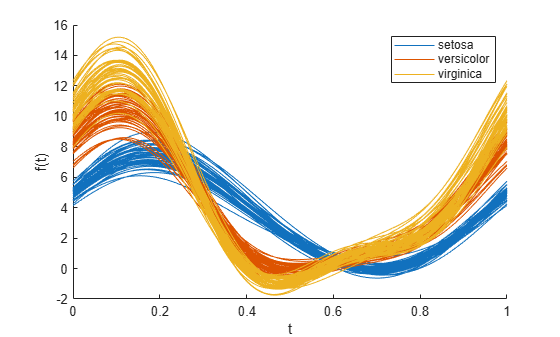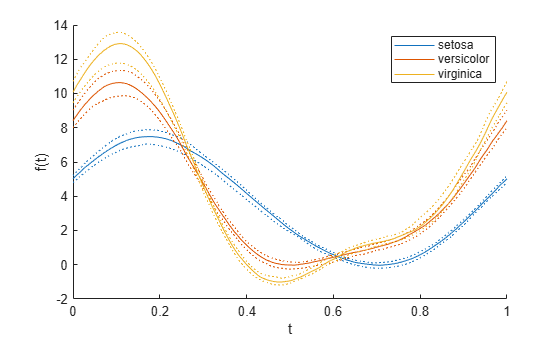andrewsplot

Syntax

andrewsplot(X)
andrewsplot(X,...,'Standardize',standopt)
andrewsplot(X,...,'Quantile',alpha)
andrewsplot(X,...,'Group',group)
andrewsplot(X,...,'PropName',PropVal,...)
andrewsplot(ax,X,...)
h = andrewsplot(X,...)

Description

andrewsplot(X) creates an Andrews plot of the multivariate data in the matrix X. The rows of X correspond to observations, the columns to variables. Andrews plots represent each observation by a function f(t) of a continuous dummy variable t over the interval [0,1]. f(t) is defined for the ith observation in X as

$f\left(t\right)=X\left(i,1\right)/\sqrt{2}+X\left(i,2\right)\mathrm{sin}\left(2\pi t\right)+X\left(i,3\right)\mathrm{cos}\left(2\pi t\right)+\dots$

andrewsplot treats NaN values in X as missing values and ignores the corresponding rows.

andrewsplot(X,...,'Standardize',standopt) creates an Andrews plot where standopt is one of the following:

• 'on' — scales each column of X to have mean 0 and standard deviation 1 before making the plot.

• 'PCA' — creates an Andrews plot from the principal component scores of X, in order of decreasing eigenvalue. (See pca.)

• 'PCAStd' — creates an Andrews plot using the standardized principal component scores. (See pca.)

andrewsplot(X,...,'Quantile',alpha) plots only the median and the alpha and (1 – alpha) quantiles of f(t) at each value of t. This is useful if X contains many observations.

andrewsplot(X,...,'Group',group) plots the data in different groups with different colors. Groups are defined by group, a numeric array containing a group index for each observation. group can also be a categorical array, character matrix, string array, or cell array of character vectors containing a group name for each observation.

andrewsplot(X,...,'PropName',PropVal,...) sets optional Line object properties to the specified values for all Line objects created by andrewsplot. (See Line Properties.)

andrewsplot(ax,X,...) uses the plot axes specified in ax, an Axes object. (See axes.) Specify ax as the first input argument followed by any of the input argument combinations in the previous syntaxes.

h = andrewsplot(X,...) returns a column vector of handles to the Line objects created by andrewsplot, one handle per row of X. If you use the 'Quantile' input parameter, h contains one handle for each of the three Line objects created. If you use both the 'Quantile' and the 'Group' input parameters, h contains three handles for each group.

Examples

collapse all

This example shows how to create an Andrews plot to visualize grouped sample data.

Create an Andrews plot, grouping the sample data by species.

andrewsplot(meas,'group',species)Create a second, simplified Andrews plot that only displays the median and quartiles of each group.

andrewsplot(meas,'group',species,'quantile',.25)